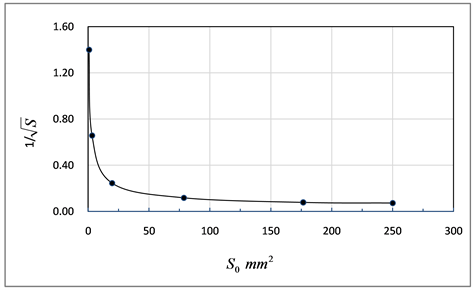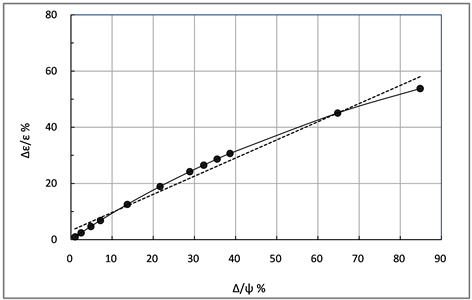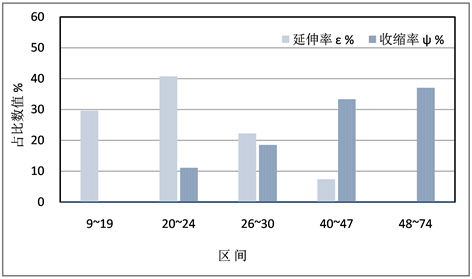# 关于收缩率和延伸率间偏差和颈缩分析IAnalysis on Deviation and Necking Formula between the Elongation & Reduction I

DOI: 10.12677/MS.2019.91009, PDF, HTML, XML, 下载: 439  浏览: 1,715  科研立项经费支持

Abstract: According to density invariability of elongation and reduction, we can deduce the compare mathematical relation of them. Equations to cause necking and deviation routes are gained. From deviation relations, we observe that in the case of smaller than 0.14 reduction deviation it will become good to compare with elongation one. In the case of deviation bigger than certain value reduction one of 0.64 will be good too. They are above 1.32 times good in this value. The maximum deviation is 1.64. (√S0)3-S√S0+S0-2S<0 etc. two formulas are the necking equation here. According to literature statistic, the reduction is more above 25% while elongation is more below 25%. The twin is caused by shear stress, and dislocation is caused by tensile stress.

1. 引言

2. 计算过程

2.1. 设$\psi =\frac{1}{1/\epsilon +1}$ (1)

$\psi =f\left(1/\sqrt{{S}_{0}}\right)$

$\psi =\frac{1}{\sqrt{{S}_{0}}+1}$ (2)

$1/\epsilon =\sqrt{{S}_{0}}$ (3)

$\epsilon =1/\sqrt{{S}_{0}}$ (4)

$\epsilon =\frac{\psi }{1-\psi }$ (5)

$\epsilon =\frac{1}{1/\psi -1}=\frac{1}{{S}_{0}/\Delta S-1}$ (6)

$\epsilon =\frac{1}{{S}_{0}/\Delta S-1}$ (7)

$\frac{1}{\sqrt{{S}_{0}}+1}\ge \frac{1}{{S}_{0}/\Delta S-1}$ (8)

 (9)

${\left(\sqrt{{S}_{0}}\right)}^{3}-S\sqrt{{S}_{0}}+{S}_{0}-2S<0$ (10)

$\frac{\text{d}\left(1/\sqrt{y}\right)}{\text{d}x}<0$ (11)

$S>\frac{{\left(\sqrt{{S}_{0}}\right)}^{3}+{S}_{0}}{2+\sqrt{{S}_{0}}}$ (12)

2.2. 设 $\epsilon =\sqrt{{S}_{0}}$

$\psi = f\left(\sqrt{{S}_{0}}\right)$

$\psi =\frac{1}{1/\sqrt{{S}_{0}}+1}$ (13)

$\epsilon =\sqrt{{S}_{0}}$ (14)

$\epsilon =\frac{\psi }{1-\psi }$ (15)

$\epsilon =\frac{1}{1/\psi -1}=\frac{1}{{S}_{0}/\Delta S-1}$ (16)

$\epsilon =\frac{1}{{S}_{0}/\Delta S-1}$ (17)

$\frac{1}{1/\sqrt{{S}_{0}}+1}\ge \frac{1}{{S}_{0}/\Delta S-1}$ (18)

${S}_{0}/\Delta S-1/\sqrt{{S}_{0}}\ge 2$ (19)

${S}_{0}\sqrt{{S}_{0}}-2S\sqrt{{S}_{0}}+{S}_{0}-S<0$ (20)

$S\ge \frac{{S}_{0}\left(1+\sqrt{{S}_{0}}\right)}{2\sqrt{{S}_{0}}+1}$ (21)

3. 讨论与结论

${S}_{0}$ 的关系曲线如图1$1/\sqrt{S}$ 随着 ${S}_{0}$ 从0到20mm2增大而变小，它从1.5急剧减少到0.3，计算得知S从0.44猛增到11 mm2。在初始面积为10 mm之后 $1/\sqrt{S}$ 缓慢降低，之后 $1/\sqrt{S}$ 变平稳。因此选择试件时要注意 ${S}_{0}$ 的尺寸不宜过小，S将变小。在曲线上方为颈缩发生区域，越往上颈缩越大。初始横截面 ${S}_{0}$ 越小颈缩越不易发生。上述两种情况的函数曲线差不多。都是下端趋于无限大。Figure 1. Relation between the cross after failure and initial cross when $\epsilon =1/\sqrt{{S}_{0}}$Figure 2. Relation between the true elongation deviation and the reduction deviationTable 1. Deviation of reduction between ψt = 1.14ψ and ψt = 1.64ψFigure 3. Relation of the reduction and elongation in literature  etc.

4. 结论

1) 根据颈缩方程式 ${\left(\sqrt{{S}_{0}}\right)}^{3}-S\sqrt{{S}_{0}}+{S}_{0}-2S<0$ 等两公式得出的曲线可知随着 ${S}_{0}$ 的增加 $1/\sqrt{S}$ 减小。

2) 收缩率的偏差在大于50%时它的偏差大于延伸率的1.32倍，说明收缩率比延伸率真实约1.32倍。与上一篇收缩率偏差大于延伸率的1.83比较小了一些，说明收缩率偏差在数值大时更大更真实。根据文献统计收缩率值在大于25%时比较多，而延伸率则在低于此值较多。收缩率大概为延伸率的0.5到2倍以上。根据曲线偏差最大值为1.64。

KOSEF (the Korea of Science and Engineering Fund) under the Specified base program 96-0300-11-01-3。

  王天宏, 吴善辛, 丁勇. 材料力学实验指导书[M]. 北京: 中国水利水电出版社, 2016: 14.  许润, 林水根. 关于拉伸试验中真实收缩率和延伸率间偏差和颈缩分析[J]. 材料科学, 2018, 8(11): 1029. https://doi.org/10.12677/MS.2018.811122  刘炳广, 陆恒昌, 麻永林, 等. Q345D的高温力学性能实验研究[J]. 内蒙古科技大学学报, 2013, 32(2): 120.